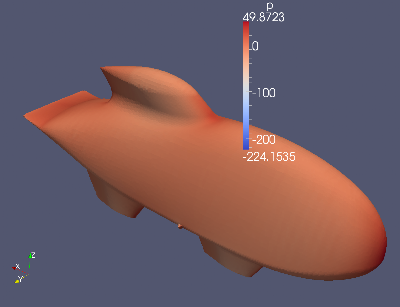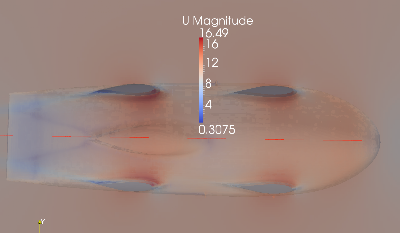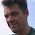## Tuesday, April 17, 2012

### First run done

This morning the first calculation was ready. I used the simpleFoam solver with a k-omega model.streamlines and pressure on the spats
Here an expert (not me) can evaluate the convergence
Time = 403
smoothSolver:  Solving for Ux, Initial residual = 5.14663e-05, Final residual = 4.49128e-06, No Iterations 3
smoothSolver:  Solving for Uy, Initial residual = 0.000128727, Final residual = 1.06587e-05, No Iterations 3
smoothSolver:  Solving for Uz, Initial residual = 7.15543e-05, Final residual = 5.27129e-06, No Iterations 3
GAMG:  Solving for p, Initial residual = 0.000129478, Final residual = 6.41391e-06, No Iterations 2
time step continuity errors : sum local = 3.84464e-10, global = 1.62637e-11, cumulative = 7.5432e-07
smoothSolver:  Solving for omega, Initial residual = 5.26723e-07, Final residual = 3.78036e-08, No Iterations 3
smoothSolver:  Solving for k, Initial residual = 3.84651e-06, Final residual = 2.74064e-07, No Iterations 3
ExecutionTime = 10837.4 s  ClockTime = 11064 s

I learned that CFD people usually use a reduced pressure: p/rho. This originates from Bernoulli: the dynamic pressure p = rho/2*v^2. p/rho equals 1/2*v^2. I set the air density rho to 1.2 [kgm^-3]. This would mean that the pressure range is -226*1.2 to 50*1.2, -271 to 60 [Pa]. Atmospheric pressure is 1e5 [Pa]. In the CFD only relative pressures are calculated. (I found a declaration of pressure in a file initialConditions somewhere, it was set to 0). The lowest pressure is 1e5 -271 [Pa], the highest 1e5 +60 Pa. The pressure change is very small relative to the atmospheric pressure. ~200/1e5=2e-3=0.002 (0.2 %). Please correct me if I'm wrong.Pressure on the body. p/rho [m^2/s^2]Something went wrong. Is is the mesh or is it paraview ?The bottom view shows low pressure spots. p/rho [m^2s^-2]Air speed [m/s] in a slice of the air 60 mm above the ground. (vehicle speed is 10m/s)The pressure distribution in mid plane p/rho [m^2s^-2]

The air is moving through the narrow space between wheel 'pants' bottom and road. In this narrow space the air velocity rises and pressure falls.

Now I would like to find out:
1. are my statements about the pressure above correct?
2. is there something wrong with the mesh?
3. how do I check the quality of the simulation using the lines on Final residual?
4. how do I calculate the forces on the body due to kinematic pressure and viscosity (skin friction)?
5. in the boundary layer what is the type of flow: laminar or turbulent?
6. I found out that the mesh is in [mm]. Both in the blockMeshDict and the snappyHexMeshDict the geometry is in mm. But shouldn't it be in [meter]. I know I can use to keyword convertToMeter in  blockMeshDict but what about the snappyHexMeshDict?
I hope someone is willing to help me on this.

1.Hi,

1) yes, the relative pressure does not change much judging from your results.

2) It is impossible to judge the quality of the mesh without having it. In Fig. 2 you say "Something went wrong. Is is the mesh or is it paraview ?". What are you referring to?

3) Residuals should flatten when the simulation is converged. Hint: set a very low tolerance for all the variables (something like 1.0e-12), and perform 1000 iterations, storing the output in a log file. Then you can plot the residuals using either foamLog or the external utility pyFoam (see wiki).

4) Some forces (drag, lift) can be computed with functionObjects http://cfdcomputing.com/documents/drag_openfoam.htm

6) You can scale the STL file with

surfaceConvert input.stl output.stl -clean -scale 0.001

2.ad 2) Thre is a pimple on the body that should not be there at all

ad 6) Where should surfaceConvert input.stl output.stl -clean -scale 0.001 be placed in the snappyHexMeshDict?